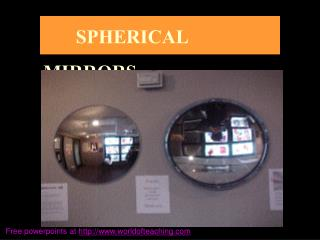DownloadDownload PresentationSPHERICAL MIRRORS

# SPHERICAL MIRRORS

Download Presentation## SPHERICAL MIRRORS

- - - - - - - - - - - - - - - - - - - - - - - - - - - E N D - - - - - - - - - - - - - - - - - - - - - - - - - - -
##### Presentation Transcript

1. SPHERICAL MIRRORS Free powerpoints at http://www.worldofteaching.com

2. What is a spherical mirror? Spherical mirrors are mirrors whose surfaces form a part of a hollow sphere, The spheres can be made of glass or any polished metal surfaces Types of spherical mirrors : 1. Convex Mirror 2. Concave Mirror

3. Convex mirror :. If the mirror coating is in the inside of the spherical surface, then the mirror is called a convex mirror Concave mirror :If the mirror coating is on the outside of the spherical surface, then the mirror is called a concave mirror.

4. Some definitions of convex and concave mirrors • 1.Center of curvature : The center of curvature of the mirror is defined as the center of the hollow sphere from which the mirror has been cut. • It is represented by the letter C. • It can be observed that C of a convex mirror is behind • the mirrored surface. • On the other hand, C for a concave mirror is in front • of the mirror.

5. Some definitions of convex and concave mirrors 2. Radius of curvature : Radius of curvature is the radius of the sphere from which the mirrors (either convex or concave) is made. It is represented by the letter R. In the figure, the distance CP is the radius of curvatures of the two mirrors.

6. Some definitions of convex and concave mirrors 3.Pole of the mirror : The central point on the surface of the mirrors is called the pole of the mirror. It is represented by the letter P. A pole of the mirror can be found by drawing a straight line from the center C to the largest distance on the surface from C. Where the line cuts the mirror, you will find the pole P

7. Some definitions of convex and concave mirrors • 4.Principal axis of the mirror : • A straight line that passes through the center of curvature C and the pole P of the mirror, is called the principal axis of the mirror. • In the figure line XY is the principal axis of the mirrors. • The principal axis of a spherical mirror, functions similar to the normal of the plane mirror. • A ray of light passing along the principal axis will be reflected back along the same path.

8. Some definitions of convex and concave mirrors • 5.Aperture of the mirror : • The portion of the hollow spherical surface from which the mirror reflections take place is called the aperture of the mirror. • In the figure 2, the distance MM’ is the aperture of the mirror.

9. Some definitions of convex and concave mirrors • 6.Focal Length of a mirror : • When parallel rays of light coming from an infinite distance, fall on either a concave or a convex mirror, they are reflected back to a point on the principal axis XY. • The focus is where the image is formed of an object placed at a far distance.

10. Focal length of a concave mirror : In a concave mirror, the rays appear to converge at a point F. The distance PF is called the focal length and F is called the focal point. The focus is in front of the mirror.

11. Focal length of a concave mirror :- In case of convex mirrors, the parallel rays, on reflection, appear to diverge from a point F. This point again is called the focal point of the convex mirrors and the distance PF is called the focal length. The focus, in this case is behind the mirror

12. Real and Virtual images 1. In a concave mirror, the focus is real. The object and the image lie on the same side of the mirrored surface. A real image is called so because it can be caught on a screen. 2. In a convex mirror, on the other hand, the object and its image lie on the opposite sides of the mirrored surface. The image therefore is a virtual image. It cannot be captured on a screen. 3. In a plane mirror, the image again is a virtual one, as it does not form on the same side as that of the object.